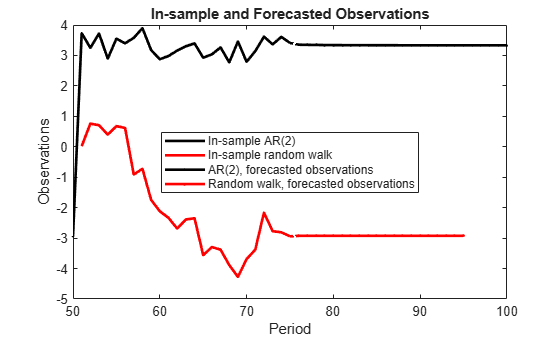Main Content

# Forecast State-Space Model Containing Regime Change in the Forecast Horizon

This example shows how to forecast a time-varying, state-space model, in which there is a regime change in the forecast horizon.

Suppose that you observed a multivariate process for 75 periods, and you want to forecast the process 25 periods into the future. Also, suppose that you can specify the process as a state-space model. For periods 1 through 50, the state-space model has one state: a stationary AR(2) model with a constant term. At period 51, the state-space model includes a random walk. The states are observed unbiasedly, but with additive measurement error. Symbolically, the model is

`$\begin{array}{l}\left[\begin{array}{c}{x}_{1,t}\\ {x}_{2,t}\\ {x}_{3,t}\\ {x}_{4,t}\end{array}\right]=\left[\begin{array}{cccc}0.5& -0.2& 1& 0\\ 1& 0& 0& 0\\ 0& 0& 1& 0\\ 0& 0& 0& 1\end{array}\right]\left[\begin{array}{c}{x}_{1,t-1}\\ {x}_{2,t-1}\\ {x}_{3,t-1}\\ {x}_{4,t-1}\end{array}\right]+\left[\begin{array}{cc}0.1& 0\\ 0& 0\\ 0& 0\\ 0& 0.5\end{array}\right]\left[\begin{array}{c}{u}_{1,t}\\ {u}_{2,t}\end{array}\right]\\ {y}_{t}=\left[\begin{array}{cccc}1& 0& 0& 0\\ 0& 0& 0& 1\end{array}\right]\left[\begin{array}{c}{x}_{1,t}\\ {x}_{2,t}\\ {x}_{3,t}\\ {x}_{4,t}\end{array}\right]+\left[\begin{array}{cc}0.3& 0\\ 0& 0.2\end{array}\right]\left[\begin{array}{c}{\epsilon }_{1,t}\\ {\epsilon }_{2,t}\end{array}\right]\end{array}.$`

For periods 1 through 50, the random walk process is not in the model.

Specify the in-sample, coefficient matrices.

```A1 = {[0.5 0.2 1; 1 0 0; 0 0 1]}; % A for periods 1 - 50 A2 = {[0.5 0.2 1; 1 0 0; 0 0 1; 0 0 0]}; % A for period 51 A3 = {[0.5 0.2 1 0; 1 0 0 0; 0 0 1 0; 0 0 0 1]}; % A for periods 51 - 75 A = [repmat(A1,50,1); A2; repmat(A3,24,1)]; B1 = {[0.1; 0; 0]}; % B for periods 1 - 50 B3 = {[0.1 0; 0 0; 0 0; 0 0.5]}; % B for periods 51 - 75 B = [repmat(B1,50,1); repmat(B3,25,1)]; C1 = {[1 0 0]}; % C for periods 1 - 50 C3 = {[1 0 0 0; 0 0 0 1]}; % C for periods 51 - 75 C = [repmat(C1,50,1); repmat(C3,25,1)]; D1 = {0.3}; % D for periods 1 - 50 D3 = {[0.3 0; 0 0.2]}; % D for periods 51 - 75 D = [repmat(D1,50,1); repmat(D3,25,1)];```

Specify the state space model, and the initial state means and covariance matrix. It is best practice to specify the types of each state using the `'StateType'` name-value pair argument. Only specify the initial state parameters for the three states that start the state-space model.

```Mean0 = [1/(1-0.5-0.2); 1/(1-0.5-0.2); 1]; Cov0 = [0.02 0.01 0; 0.01 0.02 0; 0 0 0]; StateType = [0; 0; 1]; Mdl = ssm(A,B,C,D,'Mean0',Mean0,'Cov0',Cov0,'StateType',StateType)```
```Mdl = State-space model type: ssm State vector length: Time-varying Observation vector length: Time-varying State disturbance vector length: Time-varying Observation innovation vector length: Time-varying Sample size supported by model: 75 State variables: x1, x2,... State disturbances: u1, u2,... Observation series: y1, y2,... Observation innovations: e1, e2,... State equations of period 1, 2, 3,..., 50: x1(t) = (0.50)x1(t-1) + (0.20)x2(t-1) + x3(t-1) + (0.10)u1(t) x2(t) = x1(t-1) x3(t) = x3(t-1) State equations of period 51: x1(t) = (0.50)x1(t-1) + (0.20)x2(t-1) + x3(t-1) + (0.10)u1(t) x2(t) = x1(t-1) x3(t) = x3(t-1) x4(t) = (0.50)u2(t) State equations of period 52, 53, 54,..., 75: x1(t) = (0.50)x1(t-1) + (0.20)x2(t-1) + x3(t-1) + (0.10)u1(t) x2(t) = x1(t-1) x3(t) = x3(t-1) x4(t) = x4(t-1) + (0.50)u2(t) Observation equation of period 1, 2, 3,..., 50: y1(t) = x1(t) + (0.30)e1(t) Observation equations of period 51, 52, 53,..., 75: y1(t) = x1(t) + (0.30)e1(t) y2(t) = x4(t) + (0.20)e2(t) Initial state distribution: Initial state means x1 x2 x3 3.33 3.33 1 Initial state covariance matrix x1 x2 x3 x1 0.02 0.01 0 x2 0.01 0.02 0 x3 0 0 0 State types x1 x2 x3 Stationary Stationary Constant ```

`Mdl` is a time-varying, `ssm` model without unknown parameters. The software sets initial state means and covariance values based on the type of state.

Simulate 75 observations from `Mdl`.

```rng(1); % For reproducibility Y = simulate(Mdl,75);```

`y` is a 75-by-1 cell vector. Cells 1 through 50 contain scalars, and cells 51 through 75 contain 2-by-1 numeric vectors. Cell j corresponds to the observations of period j, specified by the observation model.

Plot the simulated responses.

```y1 = cell2mat(Y(51:75)); % Observations for periods 1 - 50 d1 = cell2mat(Y(51:75)); y2 = [d1(((1:25)*2)-1) d1((1:25)*2)]; % Observations for periods 51 - 75 figure plot(1:75,[y1;y2(:,1)],'-k',1:75,[nan(50,1);y2(:,2)],'-r','LineWidth',2') title('In-sample Observations') ylabel('Observations') xlabel('Period') legend({'AR(2)','Random walk'})```Suppose that the random walk process drops out of the state space in the 20th period of the forecast horizon.

Specify the coefficient matrices for the forecast period.

```A4 = {[0.5 0.2 1 0; 1 0 0 0; 0 0 1 0; 0 0 0 1]}; % A for periods 76 - 95 A5 = {[0.5 0.2 1 0; 1 0 0 0; 0 0 1 0]}; % A for period 96 A6 = {[0.5 0.2 1; 1 0 0; 0 0 1]}; % A for periods 97 - 100 fhA = [repmat(A4,20,1); A5; repmat(A6,4,1)]; B4 = {[0.1 0; 0 0; 0 0; 0 0.5]}; % B for periods 76 - 95 B6 = {[0.1; 0; 0]}; % B for periods 96 - 100 fhB = [repmat(B4,20,1); repmat(B6,5,1)]; C4 = {[1 0 0 0; 0 0 0 1]}; % C for periods 76 - 95 C6 = {[1 0 0]}; % C for periods 96 - 100 fhC = [repmat(C4,20,1); repmat(C6,5,1)]; D4 = {[0.3 0; 0 0.2]}; % D for periods 76 - 95 D6 = {0.3}; % D for periods 96 - 100 fhD = [repmat(D4,20,1); repmat(D6,5,1)];```

Forecast observations over the forecast horizon.

`FY = forecast(Mdl,25,Y,'A',fhA,'B',fhB,'C',fhC,'D',fhD);`

`FY` is a 25-by-1 cell vector. Cells 1 through 20 contain 2-by-1 numeric vectors, and cells 51 through 75 contain scalars. Cell j corresponds to the observations of period j, specified by the forecast-horizon, observation model.

Plot the forecasts with the in-sample observations.

```d2 = cell2mat(FY(1:20)); FY1 = [d2(((1:20)*2)-1) d2((1:20)*2)]; % Forecasts for periods 76 - 95 FY2 = cell2mat(FY(21:25)); % Forecasts for periods 96 - 100 figure plot(1:75,[y1;y2(:,1)],'-k',1:75,[nan(50,1);y2(:,2)],'-r',... 76:100,[FY1(:,1); FY2],'.-k',76:100,[FY1(:,2); nan(5,1)],'.-r',... 75:76,[y2(end,1) FY1(1,1)],':k',75:76,[y2(end,2) FY1(1,2)],':r',... 'LineWidth',2') title('In-sample and Forecasted Observations') ylabel('Observations') xlabel('Period') xlim([50,100]) legend({'In-sample AR(2)','In-sample random walk',... 'AR(2), forecasted observations',... 'Random walk, forecasted observations'},'Location','Best')%% Title```Download ebook